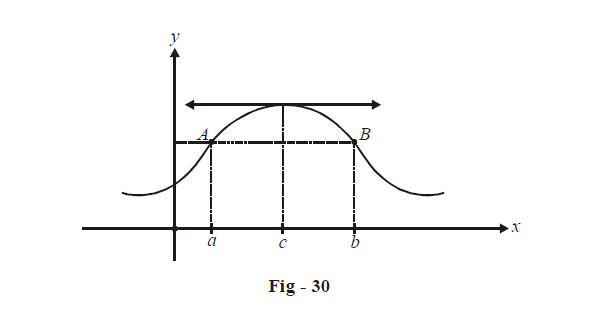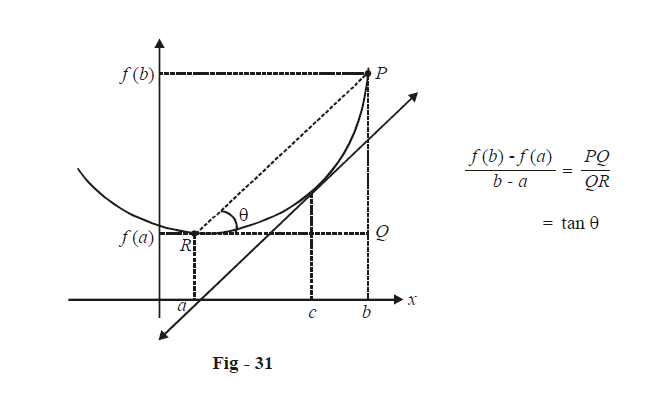# Rolles Theorem and Lagranges Theorem

Go back to  'Applications of Derivatives'

In this section we will deal with some straight forward but quite useful applications of derivatives. We start with the Rolle’s theorem, a simple but powerful theorem having a lot of practical importance.

(A)  ROLLE’S THEOREM

Let $$f\left( x \right)$$ be a function defined on [a, b] such that

(i) it is continuous on [a, b]

(ii) it is differentiable on (a, b)

(iii) f (a) = f (b)

Then there exists a real number $$c \in \left( {a,b} \right)$$  such that f '(c) = 0.

The geometrical interpretation of this theorem is quite straightforward. Consider an arbitrary curve y = f (x) and two points x = a and x = b such that f (a) = f (b).Since A and B are joined by a continuous and differentiable curve, at least one point x = c will always exist in (a, b) where the tangent drawn is horizontal, or equivalently, f '(c) = 0. Convince yourself that no matter what curve joins A and B, as long as it is continuous and differentiable one such c will always exist.

From Rolle’s theorem, it follows that between any two roots of a polynomial f (x) will lie a root of the polynomial f '(x).

The (straightforward) proof of Rolle’s theorem is left as an exercise to the reader.

(B)  LAGRANGE’S MEAN VALUE THEOREM

Let f (x) be a function defined on [a, b] such that

(i)  it is continuous on [a, b]

(ii)  it is differentiable on (a, b).

Then there exists a real number $$c \in \left( {a,b} \right)$$ such that

$f'\left( c \right) = \frac{{f\left( b \right) - f\left( a \right)}}{{b - a}}$

To interpret this theorem geometrically, we take an arbitrary function y = f (x) and two arbitrary points x = a and x = b on itWe see that no matter what the curve between R and P is like, as long as it is continuous and differentiable, there will exist a $$c \in \left( {a,\,b} \right)$$ such that the tangent drawn at x = c will have a slope equal to tan $$\theta$$ i.e, the average slope from x = a to x = b.

For a rigorous proof of LMVT, consider the function

$g\left( x \right) = f\left( x \right) - \left( {\frac{{f\left( b \right) - f\left( a \right)}}{{b - a}}} \right)x$

Verify that g(x) satisfies all the three criteria of Rolle’s theorem on [a, b] so that

$$\qquad g'\left( c \right) = 0$$ for at least one $$c \in \left( {a,\,b} \right)$$

or         \begin{align}\qquad f'\left( c \right) = \frac{{f\left( b \right) - f\left( a \right)}}{{b - a}}\end{align} for at least one $$c \in \left( {a,b} \right)$$

Notice that LMVT is an extension of the Rolle’s theorem. In fact, for f (a) = f (b), LMVT reduces  to the Rolle’s theorem.